## 1. Bloch theorem

Let’s start with the Kohn-Sham (KS) equation which has the form of Schrödinger equation for non-interacting electrons.

(1)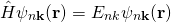where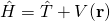. In the crystalline system,is the electron potential in a crystal

(2)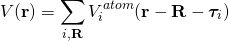where the summation runs over lattice vectors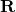and  all atomsin the unit cell.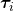is the internal coordinates of the atom in the unit cell.is the lattice vector.is a periodic function

(3)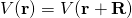According to the Bloch theorem, the KS wave function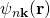has the form 

(4)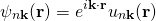In order to solve Eqn. (1), we can use the linear combination of atomic orbitals method (LCAO). Assuming

(5)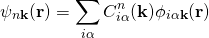where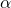is the atomic orbital index like s,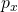,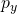,,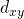,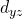,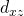,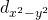,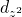, etc..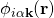is the Bloch basis. Basically, those basis are not necessary orthogonal to each other. However, here we only consider the orthogonal basis which satisfies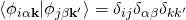There are two choices of the Bloch basisdue to the gauge freedom. The first gauge choice is

(6)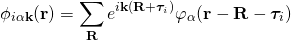where the atomic coordinatesis taken in the Fourier transform. So we name it as “atomic gauge“.

In the atomic guage, the KS equation (1) becomes

(7)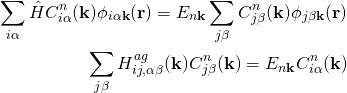Where

(8)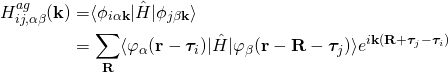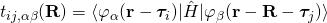are the tight-binding parameters.

The second gauge choice is

(9)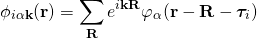where we only consider the lattice phase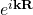contribution. So we name it as “lattice gauge“. In the lattice gauge, the KS equation is the same as the atomic gauge , however, the matric elements are different.

(10)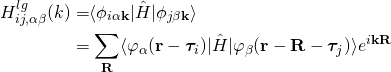Two different gauges will give you the same physics. However, the atomic gauge is more suitable for calculating properties related to the periodic part of Bloch functions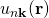. We will show that later.

### a. Periodic condition

According to the Bloch theorem, the Bloch wave function is periodic in the momentum space. i.e.

(11)where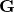is the reciprocal lattice vector satisficating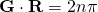. It is easy to prove that the following conditions

(12)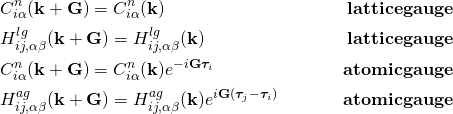## 2. Tight-binding approximation (TBA)

Actually, the formalism of the tight-binding model is listed in the above section. The spirit of TBA is by expressing the Hamiltonian by using the localized orbitals. Such localized orbitals could be atomic orbitals or Wannier functions which can be constructed from the Bloch wave function obtained from the first-principles calculations.  The TB parameters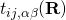can be obtained by the fitting to the DFT energy bands or directly obtained by software like Wannier90.

Once we have the TB parameters, many physical properties can be calculated. However, some properties which require the information of local coordinates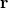can’t be obtained. Since we don’t have the exact expression of the local orbitals. In order to solve this problem, we have to build local orbitals. The simplest choice is the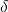function which means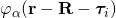is only non zero when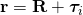. Under this basis,

(13)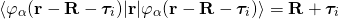## 3. The periodic part of the Bloch function

The periodic part of the Bloch function is defined as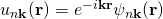.is the basis of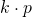model and is  used in the Berry phase, Berry curvature, shift-current, non-linear optical response and anomalous Hall conductivity calculations. In this section, we will show you that the wave function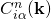obtained from the atomic guage can be directly considered as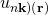in the TB formulism.

Let’s rewrite the KS equation under the basis of, then Eq.(1) becomes

(14)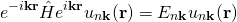Let’s define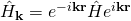. Thecan be expanded under the basis of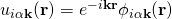since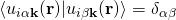at each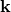point.

(15)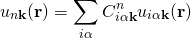Here the coefficienct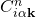is the same as Equation (5). The KS eqation becomes

(16)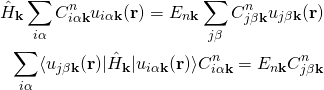We define

(17)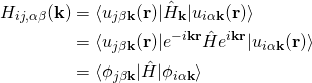It’s clearly that the matrix element is the same as that of using Bloch basis. Eventually, the eigenvectors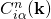are also the same with each other. If you want to use the full Bloch wave function or the periodic part of Bloch wave function, you need to take the basis into account which are shown in Eqn. (5) and (15). Let’s discuss the behavior of two gauge choices more deeply.

## 4. More about “atomic gauge” and “lattice gauge”

### Atomic gauge

The Bloch wave function is the combination of Eq.(5) and (6).

(18)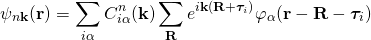Under the assumption of Eq. (13), the periodic part of the Bloch basis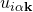becomes

(19)It’s amazing that the periodic part of the Bloch basis under the atomic gauge doesn’t dependent on the. So the k dependence of the wave function is included inwhich is element of the column vector of unitary matrix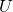which makes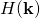(See Eqn.(17) and (8) ) diagonal

(20)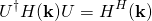Whereis a diagonal matrix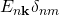, The full periodic part of the Block wave function is

(21)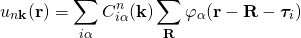So in the atomic guagecan directly be used as. For example, if we want to calculate an overlap integral betweenand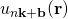, then

(22)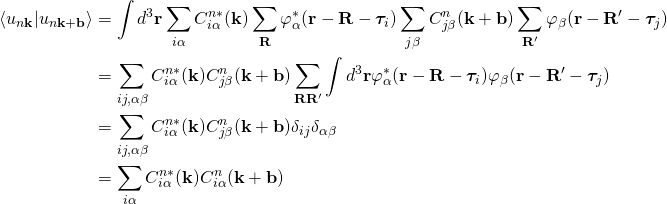While the overlap between two Bloch wave functions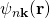and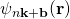(23)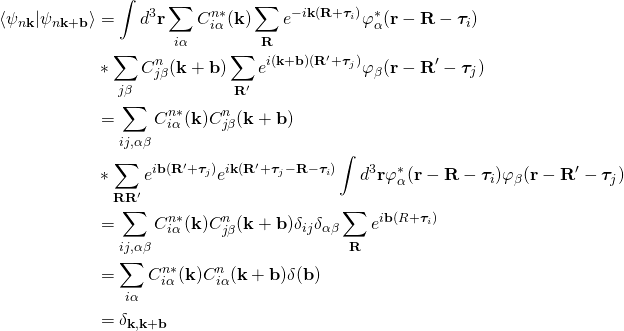It means that the Bloch wave functions is orthogonal at differentwhich is true.

### Lattice gauge

In order to get more intuition, let’s do the same thing as what we did for atomic gauge.

The Bloch wave function is the combination of Eq.(5) and (9).

(24)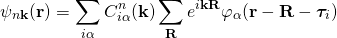Under the assumption of Eq. (13), the periodic part of the Bloch basisbecomes

(25)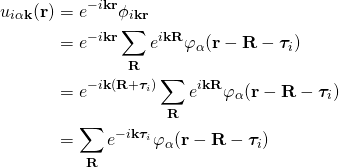Unlike the atomic gauge case, here the periodic part of the Bloch basis under the lattice gauge does dependent on the. So the k dependence of the wave function not only is included inbut also dependent on the basis. The full periodic part of the Block wave function is

(26)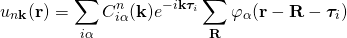Let’s calculate an overlap integral betweenand, then

(27)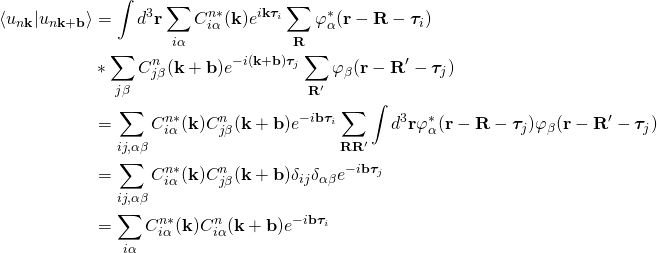While the overlap between two Bloch wave functionsand(28)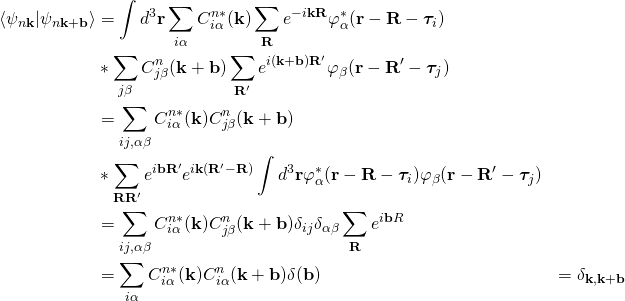It means that the Bloch wave functions is orthogonal at differentwhich is true. Here it’s worth to mention thatin the lattice gauge is not analog to the Bloch wave function, sinceare not orthogonal between two differentpoints.

 Kittel, Charles (1996). Introduction to Solid State Physics. New York: Wiley. ISBN 0-471-14286-7.

 Tight-Binding Formalism in the Context of the PythTB Package. Tahir Yusufaly, David Vanderbilt, and Sinisa Coh.

Visiting: 6,008 views